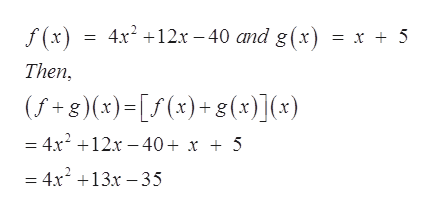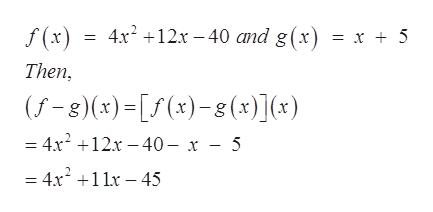Question
3 views
check_circle

Step 1

Refer to the question for the given function f(x) = 4x2+12x-40 and g(x) = x + 5. we need to calculate the (f+g)(x), (f-g)(x) , (fg)(x) and f/g (x)  and also their domain.

Then to find (f+g)(x),help_outlineImage Transcriptionclosef (x) 4x212.x -40 and g(x) = x + 5 Then, (f+8)(x)[(x)+g8(x)](x) = 4x212x-40+ x + 5 = 4x213x -35 fullscreen
Step 2

The domain of the function is that value of x for which the function is defined. And (f+g)(x) = 4x2+13x-35 is a polynomial function so it defined for all value of x.

That is,

Step 3

Similarly, for (...help_outlineImage Transcriptionclosef (x) 4x212.x -40 and g(x) = x + 5 Then, (-8)(x)[(x)-g (x)](x) = 4x212x 40- x - 5 = 4x211 -45 fullscreen

### Want to see the full answer?

See Solution

#### Want to see this answer and more?

Solutions are written by subject experts who are available 24/7. Questions are typically answered within 1 hour.*

See Solution
*Response times may vary by subject and question.
Tagged in

### Algebra